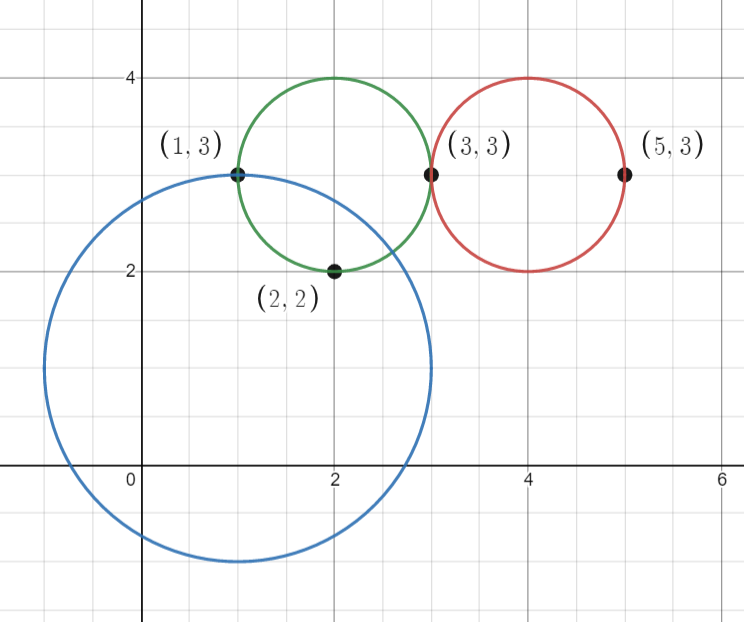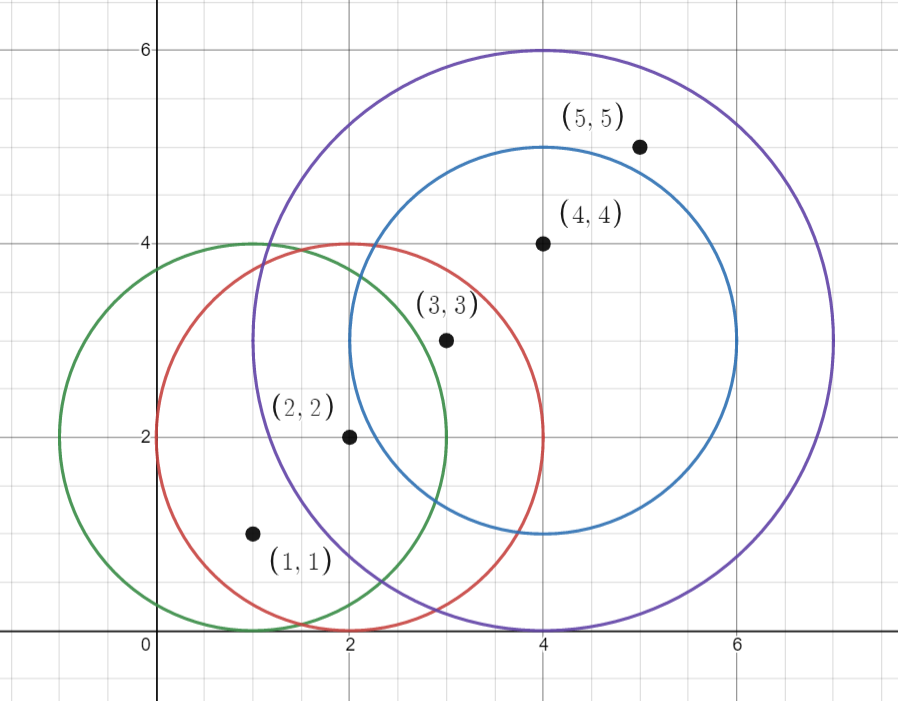1828. Queries on Number of Points Inside a Circle

#### QUESTION:

You are given an array points where points[i] = [xi, yi] is the coordinates of the ith point on a 2D plane. Multiple points can have the same coordinates.

You are also given an array queries where queries[j] = [xj, yj, rj] describes a circle centered at (xj, yj) with a radius of rj.

For each query queries[j], compute the number of points inside the jth circle. Points on the border of the circle are considered inside.

Example 1:``````Input: points = [[1,3],[3,3],[5,3],[2,2]], queries = [[2,3,1],[4,3,1],[1,1,2]]
Output: [3,2,2]
Explanation: The points and circles are shown above.
queries is the green circle, queries is the red circle, and queries is the blue circle.
``````

Example 2:``````Input: points = [[1,1],[2,2],[3,3],[4,4],[5,5]], queries = [[1,2,2],[2,2,2],[4,3,2],[4,3,3]]
Output: [2,3,2,4]
Explanation: The points and circles are shown above.
queries is green, queries is red, queries is blue, and queries is purple.
``````

Constraints:

``````1 <= points.length <= 500
points[i].length == 2
0 <= x​​​​​​i, y​​​​​​i <= 500
1 <= queries.length <= 500
queries[j].length == 3
0 <= xj, yj <= 500
1 <= rj <= 500
All coordinates are integers.
``````

Follow up: Could you find the answer for each query in better complexity than O(n)?

#### SOLUTION:

``````class Solution {
func countPoints(_ points: [[Int]], _ queries: [[Int]]) -> [Int] {
var result:[Int] = []
for query in queries {
var count = 0
for point in points {
if ((query-point)*(query-point)+((query-point)*(query-point)) <= query*query) {
count += 1
}
}
result.append(count)
}
return result
}
}
``````